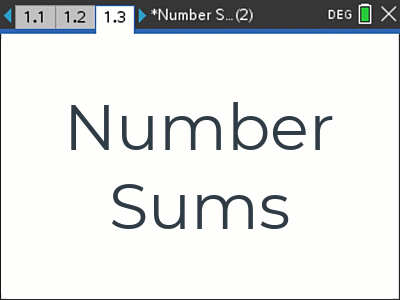# Activities

•• ##### Subject Area

• Mathematics: Algebra and Pattern: Algebra and Pattern
• Standard: National Curriculum 7-10: Number and Algebra: Linear and non-Linear Relationships
• Standard: National Curriculum 7-10: Number and Algebra: Patterns and algebra

• ##### AuthorMiddle
Aust Senior

90 Minutes

• ##### Device
• TI-Nspire™ CAS
• TI-Nspire™ Navigator™
• ##### Software

TI-Nspire™ CAS
TI-Nspire™ CAS Navigator™ NC System

3.9

## Number Sums#### Activity Overview

This activity starts with a problem to solve: Determine the number of cans in a stack, 30 rows high. Students use a range of visual and numerical representations of similar, related problems to build an understanding of associated algebraic formulas that can be used to solve this problem. The focus of the activity is on developing algebraic formulas with a fundamental understanding of their structure and therefore a deeper understanding of their meaning.

#### Objectives

Explore the connection between algebraic and graphical representations of relations such as simple quadratics, circles and exponentials using digital technology as appropriate - ACMNA239.

• Equation
• Expression
• Variable
• Sum